# Tangents of circlePage 1

#### WATCH ALL SLIDES

Slide 1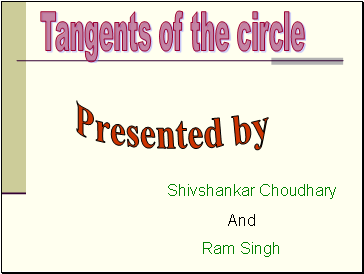## Tangents of the circle

Presented by

Shivshankar Choudhary

And

Ram Singh

Slide 2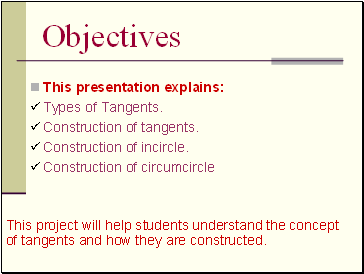## Objectives

This presentation explains:

Types of Tangents.

Construction of tangents.

Construction of incircle.

Construction of circumcircle

This project will help students understand the concept of tangents and how they are constructed.

Slide 3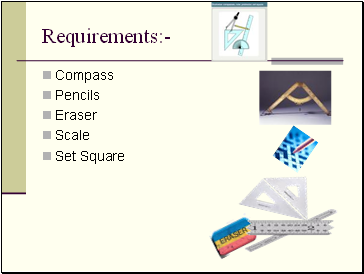## Requirements

Compass

Pencils

Eraser

Scale

Set Square

Slide 4## Tangent Chord Secent

If line touches the circle at one point only that is called a tangent

If line connect the two point at the circle that is called a chord

If line intersect the circle at two point that is called secant

Slide 5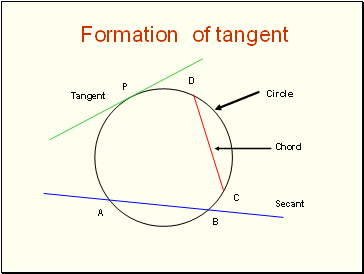## Formation of tangent

A

B

Secant

C

D

P

Tangent

Slide 6## Defination of tangents

APB is called a tangent to the circle

The touching point P is called the point of contact.

C

A

B

P

Slide 7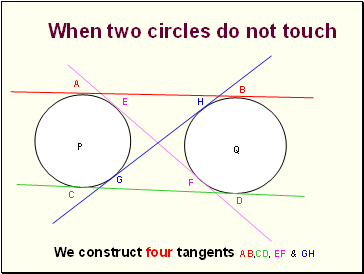A

B

C

D

E

F

G

H

P

Q

We construct four tangents AB,CD, EF & GH

When two circles do not touch

Slide 8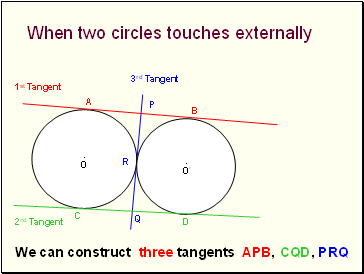A

B

C

D

O

O’

.

.

We can construct three tangents APB, CQD, PRQ

When two circles touches externally

P

Q

1st Tangent

2nd Tangent

3rd Tangent

R

Slide 9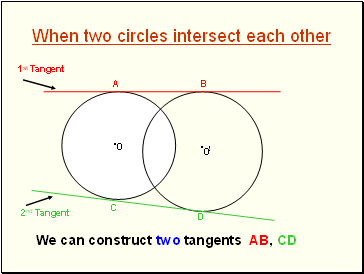When two circles intersect each other

A

B

C

D

1st Tangent

2nd Tangent

O

O

!

.

.

We can construct two tangents AB, CD

Slide 10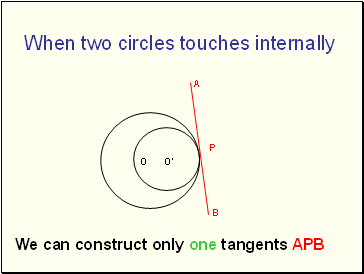A

B

O

O’

When two circles touches internally

We can construct only one tangents APB

P

Slide 11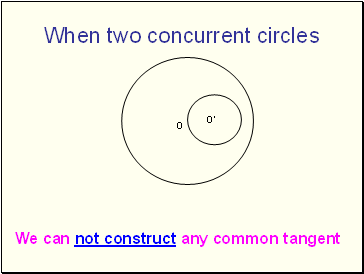When two concurrent circles

O

O’

We can not construct any common tangent

Slide 12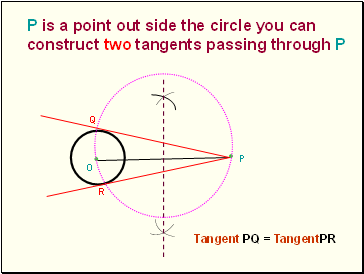P

P is a point out side the circle you can construct two tangents passing through P

O

Q

R

Tangent PQ = TangentPR

Go to page:
1  2